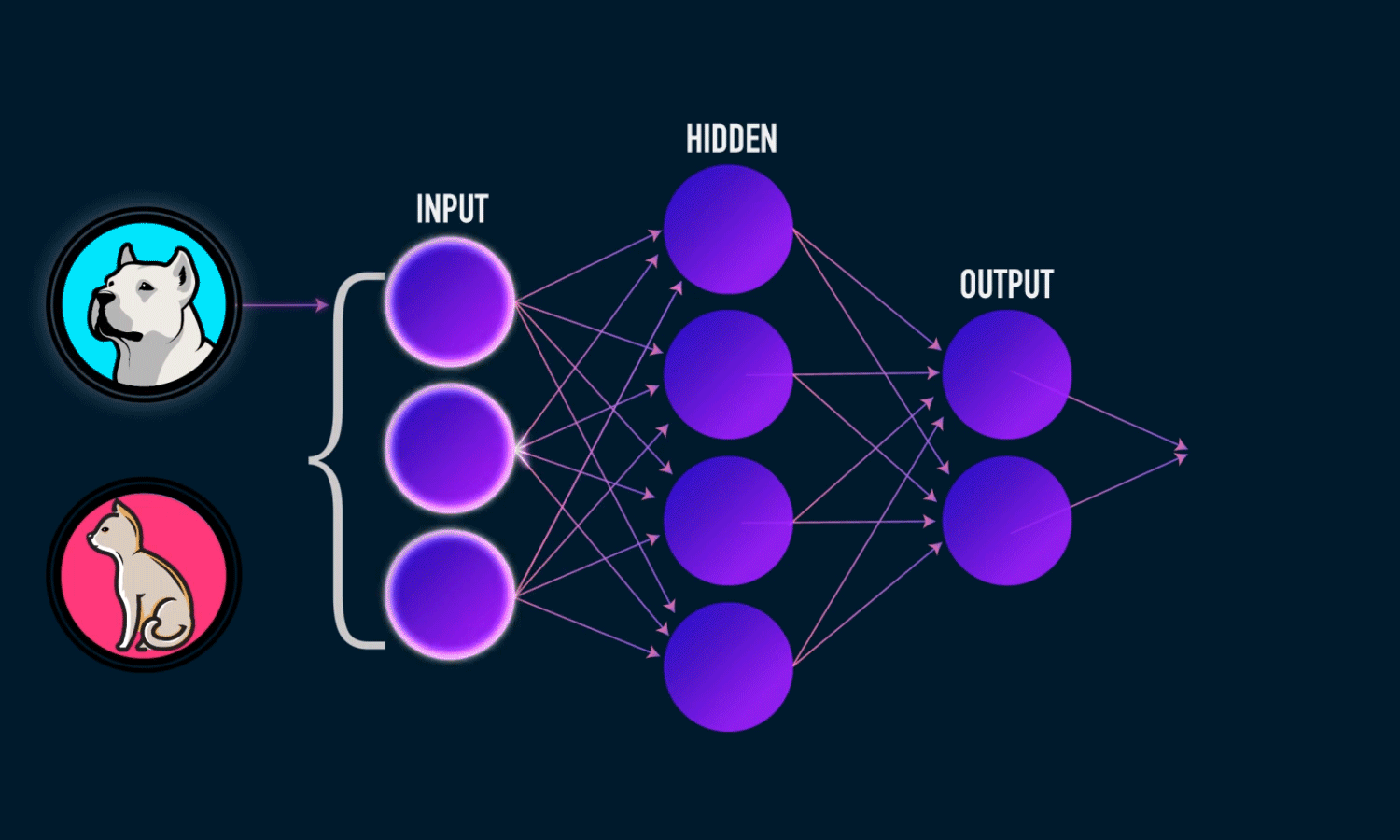# I. What is RNN?

## output_t=activation(W*input_t+U*state_t+bias)

• t is for time
• initial state can be any random vector, even zero vector.
• So, the RNN layers that we will be looking at very soon, i.e., SimpleRNN, LSTM and GRU layers follow a very similar mechanism in a sense that these RNN layers will find most adequate W’s and U’s; weights.

# II. SimpleRNN in Keras

1. SimpleRNN has 2 modes of output
• It takes inputs of 3D tensor of shape (batch_size, time_steps, input_features)
• Then, it can return a 2D tensor of shape (batch_size, output_features) which is the last output for each input sequence.
`from keras.models import Sequentialfrom keras.layers import Embedding, SimpleRNNmodel = Sequential()model.add(Embedding(10000, 32))model.add(SimpleRNN(32))model.summary()`
• Or it can return a 3D tensor of shape (batch_size, time_steps, output_features) which is the full sequences of successive outputs for each time steps by adding return_sequences=True.
`model = Sequential()model.add(Embedding(10000, 32))model.add(SimpleRNN(32,return_sequences=True))model.summary()`
• 10,000*32=320,000 ←we have done this in word-embeddings
• (32+32+1)*32=2080.
- The first 32 is from the 32-dimensional word embedding layer which will be an input for the RNN layer in each iteration. (W)
- The second 32 is the dimension of output shape in the previous time step which is defined in SimpleRNN(32). (U)
- The 1 is for the bias.
- Lastly, 32 is from the number of units which is same as the number of time steps.
`model = Sequential()model.add(Embedding(10000, 32))                #32*10,000model.add(SimpleRNN(64,return_sequences=True)) #(32+64+1)*64=6208model.add(SimpleRNN(32,return_sequences=True)) #(64+32+1)*32=3104model.add(SimpleRNN(32,return_sequences=True)) #(32+32+1)*32=2080model.summary()`
`model = Sequential()model.add(Embedding(10000, 1))                 model.add(SimpleRNN(64,return_sequences=True)) model.add(SimpleRNN(3,return_sequences=True))  model.add(SimpleRNN(2))                        model.summary()`
`from keras.datasets import imdbfrom keras.preprocessing import sequencefrom keras.layers import Densemax_features = 10000maxlen = 500batch_size = 32`
• Import data
`print('Loading data...')(input_train, y_train), (input_test, y_test) = imdb.load_data( num_words=max_features)print(len(input_train), 'train sequences')print(len(input_test), 'test sequences')print('Pad sequences (samples x time)')input_train = sequence.pad_sequences(input_train, maxlen=maxlen)input_test = sequence.pad_sequences(input_test, maxlen=maxlen)print('input_train shape:', input_train.shape)print('input_test shape:', input_test.shape)`
• Modeling
`model = Sequential()model.add(Embedding(max_features, 32)) #max_feature=10,000 so, 320,000model.add(SimpleRNN(32))               #(32+32+1)*32=2080model.add(Dense(1, activation='sigmoid'))#(32+1)*1=33model.summary()`
• Compiling and fitting
`model.compile(optimizer='rmsprop', loss='binary_crossentropy',metrics=['acc'])history = model.fit(input_train, y_train,epochs=10, batch_size=128, validation_split=0.2)#25,000*0.8=20,000 (train on 20000samples) 5000 left for validation`
• Plotting
`import matplotlib.pyplot as pltacc = history.history['acc']val_acc = history.history['val_acc']loss = history.history['loss']val_loss = history.history['val_loss']epochs = range(1, len(acc) + 1)plt.plot(epochs, acc, 'bo', label='Training acc')plt.plot(epochs, val_acc, 'b', label='Validation acc')plt.title('Training and validation accuracy')plt.legend()plt.figure()plt.plot(epochs, loss, 'bo', label='Training loss')plt.plot(epochs, val_loss, 'b', label='Validation loss')plt.title('Training and validation loss')plt.legend()plt.show()`

# III.LSTM in Keras

• Modeling
We have already imported dataset, if you are starting from this line go up and copy the parts
`from keras.layers import LSTMmodel = Sequential()model.add(Embedding(max_features, 32)) #max_features=10,000 so 320,000model.add(LSTM(32))model.add(Dense(1, activation='sigmoid'))model.summary()`
• Compiling and fitting
`model.compile(optimizer='rmsprop',loss='binary_crossentropy',              metrics=['acc'])history = model.fit(input_train, y_train,                    epochs=10,                    batch_size=128, validation_split=0.2)`
• Plotting

--

--

--

## More from A Ydobon

Ydobon is nobody.

Love podcasts or audiobooks? Learn on the go with our new app.

## Detection of Soccer Players from Thermal Images using Monk AI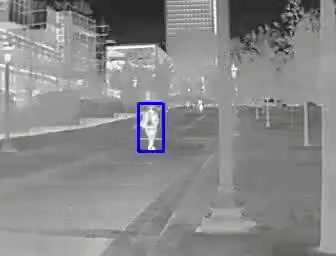## Federated Learning: A Simple Implementation of FedAvg (Federated Averaging) with PyTorch## Delivery time Prediction System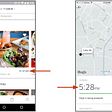## Using the latest advancements in deep learning to predict stock price movements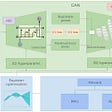## Learn linear regression with simple example.## Classical Algorithms in Force-Directed Placement## Temporal Difference Learning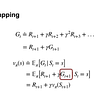## Variational Autoencoders: An Intuitive Explanation & Some Keras Code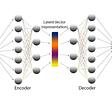## A Ydobon

Ydobon is nobody.

## Understanding Gramian Angular Field to convert your time-series data into an image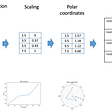## How to train AI Beast🚀## Possibilities and inabilities of Recurrent Neural Networks in NLP## Final Project: Natural Image classifier